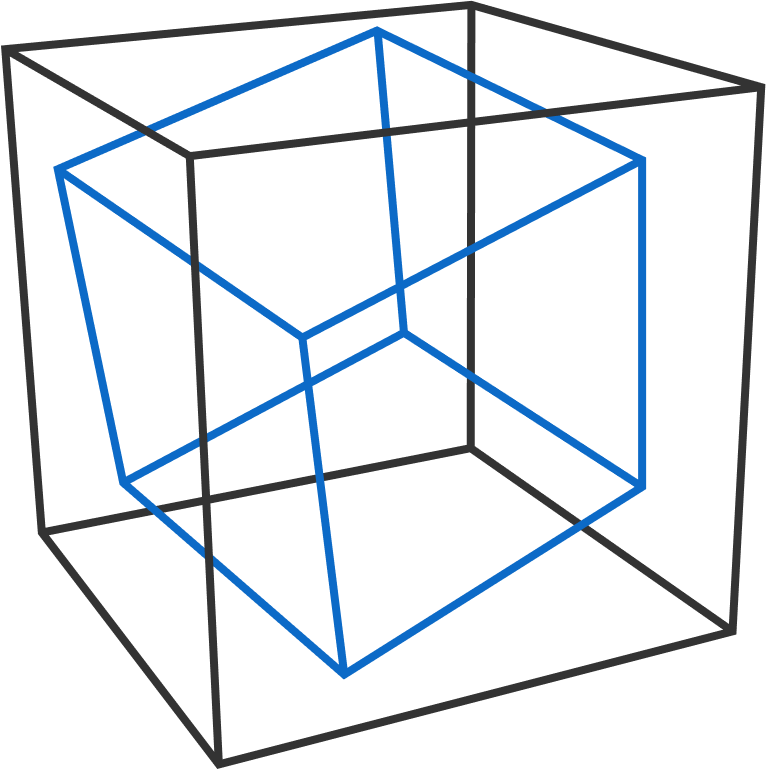Problems of the Week

Contribute a problem

A function $f(n)$ is such that $f(n)=1$ for $n<0,$ and $f(n)=1-f(n-1)f(n-3)f(n-4)$ for $n \geq 0.$

What is $\displaystyle \sum_{n=0}^{2018} f(n)?$

Bonus: What game is $f(n)$ intended to model?

The triplets of consecutive integers $(0,1,2)$ and $(1,2,3)$ have the property that the sum of their cubes is a perfect square:

$0^3 + 1^3 + 2^3 = 3^2,\quad 1^3 + 2^3 + 3^3 = 6^2 .$

Find the next smallest triplet of consecutive integers $(a, b, c)$ that has this property.

What is $a+b+c?$A cube is inscribed in a unit cube so that exactly 6 of the inscribed cube's 8 vertices each touch a different face of the unit cube.

What's the smallest cube that can be inscribed in this way?

If its side length can be expressed as $\frac{a}{b},$ where $a$ and $b$ are coprime positive integers, give the product $a \times b$ as the answer.

Three 6-sided fair dice are rolled and their sum is recorded. If there were any dice with the same face value in the roll, only those dice are thrown again and their sum is added to the previous sum. This process is iterated until all numbers are distinct in the roll.

Here is an example:

• Roll #1: $\ 5 + 5 + 5=15,$ and the three 5's are rolled again.

• Roll #2: $\ 3 + 3 + 1=7,$ and the two 3's are rolled again. (The total is 15+7=22 for now.)

• Roll #3: $\ 4 + 1 =5,$ and nothing is rolled again because 4 and 1 are distinct. (The grand total is 15+7+5=27.)

What is the expected value of the grand total?

$a,b,$ and $c$ are real constants such that there exists exactly one square whose four vertices lie on the cubic curve $y=x^3+ax^2+bx+c.$

What is the area of this square (to 3 decimal places)?

×